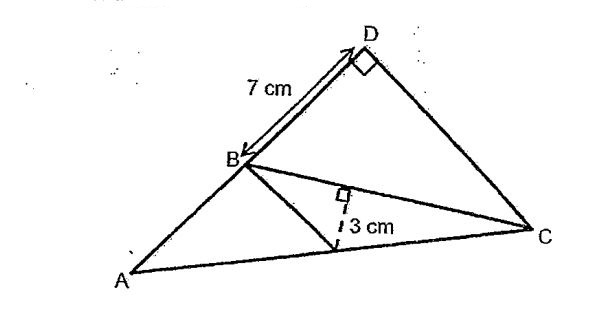# Question 1 of 31

In the figure below, AC = 15cm, CD = 9cm and AD = 12cm. What is the area of triangle ABC?A
20.5cm$^2$
B
22.5cm$^2$
C
23.5cm$^2$
D
24.5cm$^2$
E
None of the above